## Main

One way to cope with decoherence is to make use of entanglement distillation protocols by which a pure maximally entangled state may be obtained from multiple copies of partially decohered states4,10,11,12,13,14. Note, however, that it is impossible to obtain an entangled state from copies of fully decohered (that is, separable) states by applying entanglement distillation15. Another method to deal with decoherence is to rely on the so-called decoherence-free subspace16,17. The decoherence-free subspace, however, requires the interaction Hamiltonian to have an appropriate symmetry, which might not always be present. The quantum Zeno effect may also be used to suppress decoherence18,19 as well as to generate entanglement20 under some specific situations.

Our scheme for protecting entanglement from decoherence is based on the fact that weak quantum measurement can be reversed. The reversibility of weak quantum measurement was originally discussed in the context of quantum error correction21 and was demonstrated for a single superconducting qubit and a single photonic qubit22,23,24. Recently, it was shown that weak measurement and quantum measurement reversal can effectively suppress amplitude-damping decoherence for a single qubit25,26. Here, we experimentally demonstrate a scheme for protecting entanglement from amplitude-damping decoherence using weak measurement and quantum measurement reversal. The scheme can reduce or even completely nullify the effect of decoherence as evidenced by increased concurrence of the two-qubit system.

Consider a two-level quantum system (S) whose computational bases are |0〉S and |1〉S. The environment (E) is initially at |0〉E. Amplitude-damping decoherence, in which a particular computational basis state is irreversibly and probabilistically transferred to the other, results from state-dependent coupling of the system qubit to the environment and is described by the following quantum map,where 0 ≤ D ≤ 1 is the magnitude of the decoherence and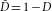(ref. 4). Amplitude-damping decoherence is highly relevant for many practical qubit systems. For instance, amplitude-damping decoherence is caused by photon loss for the vacuum–single-photon qubit, by spontaneous decay for the atomic energy level qubit and by zero-temperature energy relaxation for the superconducting qubit.

We now investigate how the decoherence map of equation (1) affects a two-qubit entangled state. In particular, we consider a quantum communication scenario depicted in Fig. 1a where Alice prepares a two-qubit entangled state |ϕ〉=α|00〉S+β|11〉S (α α*+β β*=1) and distributes the qubits to Bob and Charlie through quantum channels with decoherence D1 and D2. The discussion, however, applies equally well to other types of two-qubit entangled state and physical qubit of stationary nature.Figure 1: Scheme for protecting entanglement from decoherence using weak measurement and quantum measurement reversal.

Although the initial state was a pure two-qubit entangled state |ϕ〉, owing to decoherence, Bob and Charlie now share the two-qubit quantum state ρd given as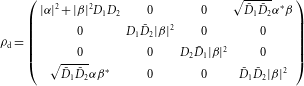where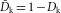( k=1,2). The effect of decoherence D1 and D2 on the initial state |ϕ〉 can then be investigated by evaluating concurrence Cd (which quantifies the amount of entanglement) of the shared quantum state ρd (ref. 27), which is calculated to be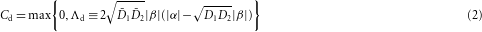Note that Cdd if Λd>0. It is clear from equation (2) that the stronger the decoherence, Dk→1 (k=1,2), the smaller the concurrence Cd. When the decoherence is strongest Dk=1 (k=1,2), concurrence Cd becomes zero, meaning that the two-qubit system has become fully separable. Furthermore, it is interesting to point out that, for a particular initial two-qubit state |ϕ〉=α|00〉S+β|11〉S with |β|≥|α|, the decoherence causes entanglement sudden death (ESD) in which Cd=0 although decoherence is not at its maximum: Cd=0 if decoherence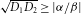(ref. 3).

Let us now describe our scheme, depicted in Fig. 1b, to protect entanglement from decoherence by making use of weak measurement and quantum measurement reversal. Before the system qubits undergo decoherence (that is, coupling to the environment), they are subject to weak measurement (Mwk), which partially collapses the state towards |0〉S (refs 22, 23, 24). The two-qubit weak measurement can be written as a non-unitary quantum operationwhere p1 and p2 are the weak measurement strengths24. As the computational basis state |0〉S does not couple to the environment as shown in equation (1), the system qubits after the weak measurement are less vulnerable to decoherence.

After the decoherence quantum channel, Bob and Charlie carry out quantum measurement reversal operations on the qubits. The two-qubit reversing measurement (Mrev) is a non-unitary operation that can be written as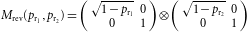where ${p}_{{\text{r}}_{\text{1}}}$ and ${p}_{{\text{r}}_{\text{2}}}$ are the strengths of the reversing measurement. The optimal reversing measurement strength that gives the maximum amount of entanglement of the two-qubit state ρr is calculated to be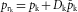, where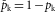( k=1,2; refs 25, 26). Assuming that the reversing measurement is optimal, the two-qubit state now shared by Bob and Charlie (after the sequence of weak measurement, decoherence and reversing measurement) is given as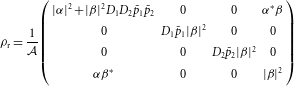where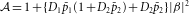. The concurrence Cr of the two-qubit state ρr shared by Bob and Charlie is then calculated to be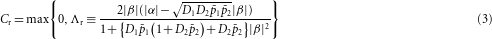where Crr if Λr>0.

We can draw two important conclusions from the result in equation (3). First, Cr is always larger than Cd, which means that weak measurement and quantum measurement reversal indeed can be used for protecting entanglement from decoherence. It is possible to achieve ρr→|ϕ〉 provided that the strength of the weak measurement pk and that of the corresponding optimal reversing measurement ${p}_{{\text{r}}_{\text{k}}}$ (k=1,2) are sufficiently strong. Second, even for the ESD condition (that is, for the initial state |ϕ〉 with |β|>|α|, Cd=0 if decoherence), by applying the entanglement protection protocol with proper weak measurement (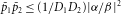) and the corresponding optimal reversing measurement, Bob and Charlie are able to share some entanglement.

In Fig. 2, we show how Cdd) and Crr) behave for two particular initial states under different decoherence, weak measurement and corresponding optimal reversing measurement. It is clear that decoherence affecting the two qubits independently and at different magnitudes can be circumvented by exploiting weak measurement and quantum measurement reversal.Figure 2: Theoretical estimation of concurrence change as functions of decoherence and weak measurement.

Let us now describe the experimental demonstration of protecting entanglement from decoherence using weak measurement and quantum measurement reversal for an entangled two-qubit system. The experimental set-up consisting of three principle sections (weak measurement, decoherence and reversing measurement) is schematically shown in Fig. 3. Details of the experimental set-up are described in the Methods section. For a clear demonstration, we consider identical decoherence and weak measurement for both qubits, that is, D1=D2=D and p1=p2=p. In our experiment, the system qubits are realized with the single-photon polarization state; |0〉S and |1〉S refer to horizontal and vertical polarization, respectively.

We first examine the effect of decoherence D on the initial two-qubit entangled state |ϕ〉=α|00〉S+β|11〉S without introducing the weak measurement and the reversing measurement. The resulting two-qubit state ρd is reconstructed with quantum state tomography24,26 and concurrence Cd is then evaluated. Figure 4a shows the experimental data for three input state conditions (|α|=|β|, |α|>|β| and |α|<|β|) as a function of decoherence D. In all cases, the data show loss of entanglement due to decoherence. Note that, for |α|<|β|, ESD occurs as expected in equation (2).Figure 4: Experimental data for protecting entanglement from decoherence using weak measurement and quantum measurement reversal.

We now test whether our entanglement protection scheme, based on weak measurement and quantum measurement reversal, can indeed circumvent decoherence. To demonstrate the scheme’s ability to protect entanglement even under severe decoherence, we chose D=0.6, at which ESD is demonstrated for the initial state with |α|<|β| as shown in Fig. 4a. Figure 4b shows concurrence Cr of the two-qubit state ρr as a function of weak measurement strength pfor two sets of initial states: |α|=|β| and |α|<|β|. For each weak measurement strength p, the optimal reversing measurement strength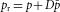was chosen. For the maximally entangled two-qubit state (|α|=|β|), the weak measurement and the reversing measurement indeed suppress two-qubit decoherence so that Cr>Cd and Cr→1 as p→1. Furthermore, as demonstrated for a non-maximally entangled state (|α|<|β|), our scheme can circumvent even ESD-causing conditions: for the weak measurement strength p larger than a certain value, the two-qubit state ρrexhibits a positive concurrence Cr, which means that Bob and Charlie can now share entanglement even through quantum channels of severe decoherence.

As the weak measurement and the reversal measurement are non-unitary operations, our scheme naturally has less than unity success probability25,26. As shown in Fig. 4b, the higher the concurrence Cr, the larger the weak measurement strength p. We can then explore the trade-off relation between the success probability and weak measurement strength p. For a single qubit, the overall success probability can be calculated by averaging the state-dependent success probability for all states on the Bloch sphere and is given by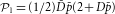(refs 25, 26). For the two-qubit state, the success probability is calculated to be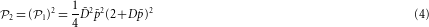We have measured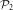by averaging the success probabilities for four two-qubit states, |00〉S, |01〉S, |10〉S and |11〉S. Owing to the symmetry, averaging over the four two-qubit states is sufficient to obtainexperimentally. Figure 5 shows the experimentally obtained success probability as a function of weak measurement strength p. Clearly, the larger the weak measurement strength p, the less the success probability. In the asymptotic limit of p→1, concurrence Cr can be arbitrarily close to the initial value but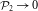.Figure 5: The success probability as a function of weak measurement strength.

We have demonstrated that weak measurement and quantum measurement reversal can indeed be useful for battling against decoherence. In particular, for amplitude-damping decoherence, we have shown that our protocol can distribute (protect) entanglement even through (from) severe decoherence. We have also studied the trade-off relation between the success probability, concurrence and weak measurement strength. Although the demonstration in this work was done for two-photon polarization qubits, the protocol can easily be applied to other types of qubit, making weak measurement and quantum measurement reversal powerful tools for battling against decoherence. We thus believe that it should be possible to effectively handle decoherence in quantum information by combining the scheme for protecting entanglement from decoherence discussed in this paper and entanglement distillation.

## Methods

### State preparation.

First, the two-qubit maximally entangled state (|ϕ〉=α|00〉S+β|11〉S with |α|=|β|) is generated using type-I frequency-degenerate spontaneous parametric down-conversion from a 6-mm-thick β-BaB2O4 crystal pumped by a 405 nm diode laser28. The photons are frequency filtered with a set of interference filters with a 5 nm passband. The non-maximally entangled states (|ϕ〉=α|00〉S+β|11〉S with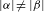) are generated by preferential amplitude reduction of one of the basis states using a set of glass plates oriented at the Brewster angle13,24,26 (BPs).

### Weak measurement and reversing measurement.

The weak measurement and the reversing measurement for the single-photon polarization qubit are implemented with BPs and wave plates24. As the BP probabilistically rejects vertical polarization (|1〉S) and completely transmits horizontal polarization (|0〉S), a single-photon polarization qubit found behind a BP had been subject to weak measurement or partial collapse measurement towards the |0〉S. The reversing measurement is designed to reverse the effect of weak measurement by making partial collapse measurement towards the |1〉S and it can be implemented by adding 45° half-wave plates (HWPs) before and after the BPs. The weak measurement and the reversing measurement strength p and pr can be varied by changing the number of BPs.

### Amplitude-damping decoherence.

The decoherence map of equation (1) causes state-dependent coupling of the system qubit (the single-photon polarization state) to the environment qubit (the single-photon path qubit) and is realized with an interferometer shown in Fig. 3 (ref. 26). The displaced Sagnac interferometer implements the coupling of the polarization qubit to the path qubit. The horizontal polarization |0〉S entering the polarizing beam splitter (PBS) can be found only at the |0〉E output mode. The vertical polarization |1〉S at the input of the PBS can be found both at |0〉E and |1〉Eoutput modes according to the angle θ of the HWP. The probability that the vertical polarization |1〉S at the input of the PBS ends up at the |1〉E output mode of the PBS corresponds to decoherence D in equation (1) such that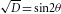. As we are interested only in the system qubit, we need to trace out the environment qubit once the coupling is done. We realize the tracing out of the environment qubit by incoherently mixing |1〉E(horizontally polarized) and |0〉E (vertically polarized) at another beam splitter (BS) with a path-length difference sufficiently larger than the coherence length (140 μm) of the single photon.